# Atomic Structure and Periodic TrendsPage 14

#### WATCH ALL SLIDES

Li(g)

Li+(g) + e-(g)

I1 = 520 kJ/mol

M+(g)

M2+(g) + e-(g)

Li+( g)

Li2+(g) + e-(g)

I2 = 7300 kJ/mol

1st IE

2nd IE

These reactions require energy (endothermic).

Periodic Trends

2) The Ionization Energies (I) within the Periodic Table

I1 = E(M+, g) – E(M, g)

I2 = E(M2+, g) – E(M+, g)

Slide 121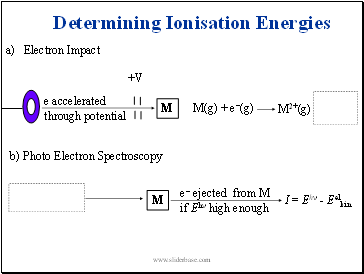Electron Impact

M

Light (Eh)

M

e- ejected from M

if Eh high enough

I = Eh - Eelkin

+V

Determining Ionisation Energies

e accelerated

through potential

b) Photo Electron Spectroscopy

M(g) + e-(g)

M2+(g) + 2 e-(g)

Slide 122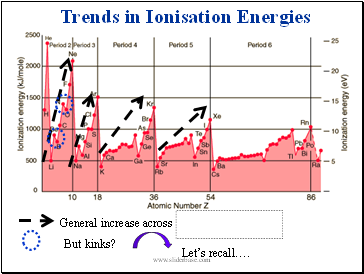Trends in Ionisation Energies

General increase across periods

But kinks?

Let’s recall….

Slide 123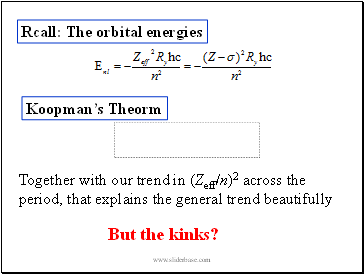Rcall: The orbital energies

Koopman’s Theorm

I  - orbital energy

Together with our trend in (Zeff/n)2 across the period, that explains the general trend beautifully

But the kinks?

Slide 124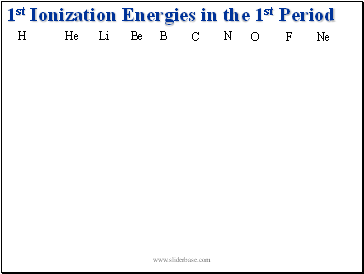1st Ionization Energies in the 1st Period

H

He

Li

Be

N

O

F

Ne

B

C

Slide 125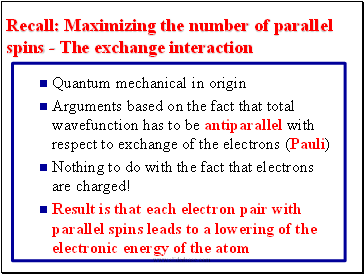Recall: Maximizing the number of parallel spins - The exchange interaction

Quantum mechanical in origin

Arguments based on the fact that total wavefunction has to be antiparallel with respect to exchange of the electrons (Pauli)

Nothing to do with the fact that electrons are charged!

Result is that each electron pair with parallel spins leads to a lowering of the electronic energy of the atom

Slide 126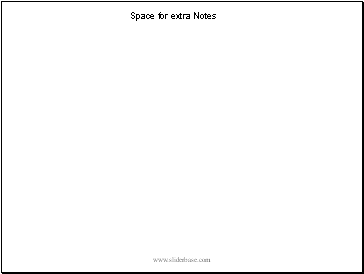## Space for extra Notes

Slide 127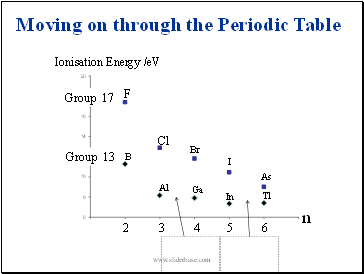B

Al

Ga

In

Tl

F

Cl

Br

Ionisation Energy /eV

2

3

4

5

6

n

Moving on through the Periodic Table

Group 13

Group 17

I

As

Slide 128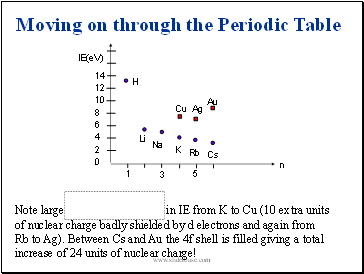Note large increase in IE from K to Cu (10 extra units of nuclear charge badly shielded by d electrons and again from Rb to Ag). Between Cs and Au the 4f shell is filled giving a total increase of 24 units of nuclear charge!

14

12

10

8

6

4

2

0

IE(eV)

H

Li

Na

K

Rb

Cs

Cu

Ag

Au

Moving on through the Periodic Table

1

3

5

n

Slide 129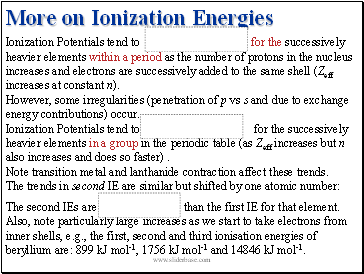## More on Ionization Energies

Go to page:
1  2  3  4  5  6  7  8  9  10  11  12  13  14  15
16  17